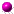Feng Kang Prize | The 2021 Winners | Entry, Rules & Contacts
Past Winners| About Feng Kang | Other Scientific Computing Contests(Feng Kang   1920-1993)

 ```On the Minimally Almost Periodic Topological Groups (pdf, 301 KB)On the Theory of Distributions (pdf, 5771KB)Duality Relations In Spaces of Distributions (pdf, 292KB)Mellin Transform In Distributions,I (pdf, 809KB)Difference Schemes Based On Variational Principle (pdf, 1217KB)The Finite Element Method (pdf, 1571KB)Elliptic Equations On Composite Manifold and Composite Elastic Structures (pdf, 395KB)On the Theory of Discontinuous Finite Elements (pdf, 377KB)Differential Versus Integral Equations and Finite Versus Infinite Elements (pdf, 305KB)Conservative Difference Method For Neutron Transport Equation and Eigenvalue Problem (pdf, 304KB)Canonical Boundary Reduction and Infinite Element Method (pdf, 475KB)Canonical Integral Equations of Elliptic Boundary-Value Problems and Their Numerical Solutions (pdf, 680KB)Finite Element Method and Natural Boundary Reduction (pdf, 423KB)Asymptotic Radiation Conditions For Reduced Wave Equation (pdf, 305KB)Some Nonlinear Problem In Modern Mathematics and Physics (pdf, 216KB)Poincare and Friedrichs Inequalities In Nonconforming Finite Element Spaces (pdf, 387KB)A Sobolev Imbedding Theorem In Nonconforming Finite Element Spaces (pdf, 84KB)Fractional Order Sobolev Spaces For Nonconforming Finite Elements (pdf, 162KB)A Theorem For the Natural Integral Operator of Harmonic Equation (pdf, 152KB)Natural Boundary Reduction and Domain Decomposition (pdf, 226KB)Inverse problems in mathematical physics (In chieses) (ps.gz file, 124 KB)On difference schemes and symplectic geometry (ps.gz file, 47KB)Difference schemes for Hamiltonian formalism and symplectic geometry (ps.gz file, 50 KB)Symplectic geometry and numerical methods in fluid dynamics (ps.gz file, 41 KB)Canonical difference schemes for Hamiltonian canonical differential equations (ps.gz file, 44 KB)The symplectic methods for the computation of Hamiltonian equations (ps.gz file, 629 KB)On the approximation of linear Hamiltonian systems (ps.gz file, 48 KB)Construction of canonical difference schemes for Hamiltonian formalism via generating functions (ps.gz file, 83 KB)Symplectic difference schemes for linear Hamiltonian canonical systems (ps.gz file, 45 KB)Hamiltonian algorithms for Hamiltonian dynamical systems (ps.gz file, 51 KB)The Hamiltonian way for computing Hamiltonian dynamics (ps.gz file, 710 KB)Hamiltonian algorithms and a comparative numerical study (ps.gz file, 994 KB)A note on conservation laws of symplectic difference schemes for Hamiltonian systems (ps.gz file, 44 KB)Symplectic difference schemes for Hamiltonian systems in general symplectic structure (ps.gz file, 53 KB)How to compute properly Newton's equation of motion ? (ps.gz file, 391 KB)Formal power series and numerical algorithms for dynamical systems (ps.gz file, 45 KB)Symplectic, contact and volume-preserving algorithms (ps.gz file, 670 KB)Formal dynamical systems and numerical algorithms (ps.gz file, 48 KB)Dynamical systems and geometric construction of algorithms (ps.gz file, 104 KB)Variations on a theme by Euler (ps.gz file, 46 KB)The step-transition operators for multi-step methods of ode's (ps.gz file, 47 KB)The calculus of generating functions and the formal energy for Hamiltonian algorithms (ps.gz file, 75 KB) ```

CONTACT:

Institute of Computational Mathematics and Scientific/Engineering Computing